Next: Example 2-d electrostatic calculation Up: Poisson's equation Previous: An example 2-d Poisson

## An example solution of Poisson's equation in 2-d

Let us now use the techniques discussed above to solve Poisson's equation in two dimensions. Suppose that the source term is(181)

forand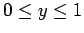. The boundary conditions at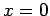are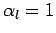,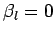, and[see Eq. (147)], whereas the boundary conditions at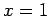are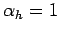,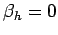, and[see Eq. (148)]. The simple Dirichlet boundary conditionsare applied atand. Of course, this problem can be solved analytically to give(182)

Figures 63 and 64 show comparisons between the analytic and finite difference solutions for. It can be seen that the finite difference solution mirrors the analytic solution almost exactly.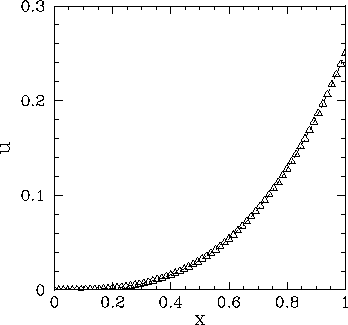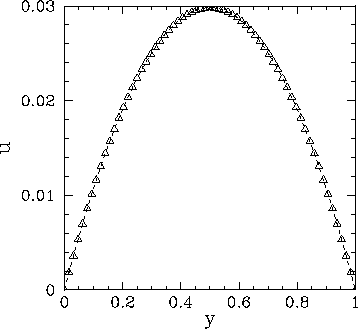As a second example, suppose that the source term is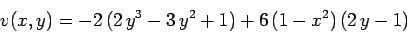(183)

forand. The boundary conditions atare,, and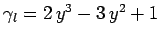[see Eq. (147)], whereas the boundary conditions atare,, and[see Eq. (148)]. The simple Neumann boundary conditionsare applied atand. Of course, this problem can be solved analytically to give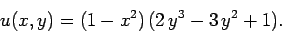(184)

Figures 65 and 66 show comparisons between the analytic and finite difference solutions for. It can be seen that the finite difference solution mirrors the analytic solution almost exactly.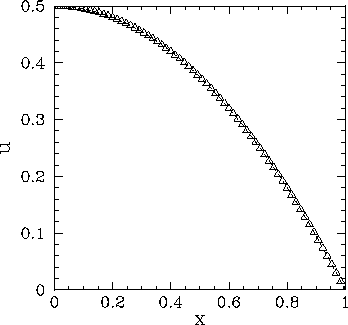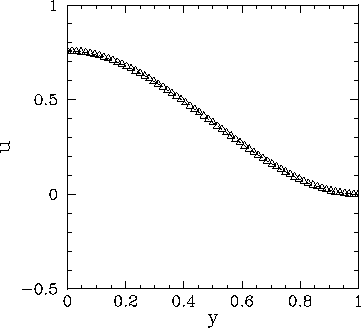Next: Example 2-d electrostatic calculation Up: Poisson's equation Previous: An example 2-d Poisson
Richard Fitzpatrick 2006-03-29